# Data Visualization with Highcharter in R

Learn how to use Highcharter to create a visualization that creates a buzz.
Aug 2018  · 12 min read

I think data visualization is the best technique to show any descriptive and analytics reporting on a chunk of data. I am a type of person who loves data visualization. You can display the whole story in a single screen well that also depends on the data complexity. If you are reading this tutorial, then I think you must be aware of the Ggplot2 package in R which is used to generate some awesome charts for analysis but somehow lacks dynamic properties.

Coming Back to Highcharter, So it is an R wrapper of HighCharts javascript library and its module.

The main features of this package are:

• You can create various charts with the same style like scatter, bubble, time series, heatmaps, treemaps, bar charts, etc.
• It supports various R objects.
• It supports Highstocks Charts, Choropleths.
• It does have a piping style which is loved by all R users and programmer.
• A large variety of themes with awesome looks.

Let's get down to the business and create some visualization with Highcharter following the features mentioned above:

## Creating some Basic Charts Using hchart Function

hchart is a generic function which takes an object and returns a highcharter object. There are functions whose behavior are similar to the functions of the ggplot2 package like:

• hchart works like ggplot2's `qplot`.
• hc_add_series works like ggplot2's `geom_S`.
• hcaes works like ggplot2's `aes`.

Let's choose a dataset. I am going to take Pokemon dataset also provided in Highcharter package. Have a glimpse of the dataset.

``glimpse(pokemon)``
``````## Observations: 718
## Variables: 20
## \$ id              <dbl> 1, 2, 3, 4, 5, 6, 7, 8, 9, 10, 11, 12, 13, 14,...
## \$ pokemon         <chr> "bulbasaur", "ivysaur", "venusaur", "charmande...
## \$ species_id      <int> 1, 2, 3, 4, 5, 6, 7, 8, 9, 10, 11, 12, 13, 14,...
## \$ height          <int> 7, 10, 20, 6, 11, 17, 5, 10, 16, 3, 7, 11, 3, ...
## \$ weight          <int> 69, 130, 1000, 85, 190, 905, 90, 225, 855, 29,...
## \$ base_experience <int> 64, 142, 236, 62, 142, 240, 63, 142, 239, 39, ...
## \$ type_1          <chr> "grass", "grass", "grass", "fire", "fire", "fi...
## \$ type_2          <chr> "poison", "poison", "poison", NA, NA, "flying"...
## \$ attack          <int> 49, 62, 82, 52, 64, 84, 48, 63, 83, 30, 20, 45...
## \$ defense         <int> 49, 63, 83, 43, 58, 78, 65, 80, 100, 35, 55, 5...
## \$ hp              <int> 45, 60, 80, 39, 58, 78, 44, 59, 79, 45, 50, 60...
## \$ special_attack  <int> 65, 80, 100, 60, 80, 109, 50, 65, 85, 20, 25, ...
## \$ special_defense <int> 65, 80, 100, 50, 65, 85, 64, 80, 105, 20, 25, ...
## \$ speed           <int> 45, 60, 80, 65, 80, 100, 43, 58, 78, 45, 30, 7...
## \$ color_1         <chr> "#78C850", "#78C850", "#78C850", "#F08030", "#...
## \$ color_2         <chr> "#A040A0", "#A040A0", "#A040A0", NA, NA, "#A89...
## \$ color_f         <chr> "#81A763", "#81A763", "#81A763", "#F08030", "#...
## \$ egg_group_1     <chr> "monster", "monster", "monster", "monster", "m...
## \$ egg_group_2     <chr> "plant", "plant", "plant", "dragon", "dragon",...
## \$ url_image       <chr> "1.png", "2.png", "3.png", "4.png", "5.png", "...``````

Let's plot a bar chart.

``````pokemon%>%
count(type_1)%>%
arrange(n)%>%
hchart(type = "bar", hcaes(x = type_1, y = n))``````So you got a bar chart concerning the type 1 category of the pokemon.

Suppose you want a column chart instead then the only variable that you need to change is the type to the column.

``````pokemon%>%
count(type_1)%>%
arrange(n)%>%
hchart(type = "column", hcaes(x = type_1, y = n))``````### Treemap

``````pokemon%>%
count(type_1)%>%
arrange(n)%>%
hchart(type = "treemap", hcaes(x = type_1, value = n, color = n))``````We can also use hc_add_series to plot the chart. It is used for adding and removing series from highchart object.### Scatterplot

``````highchart()%>%
hc_add_series(pokemon, "scatter", hcaes(x = height, y = weight))``````

The main difference in ggplot2's geom_ functions and hc_add_series is that we need to add data and aesthetics explicitly in every function while in ggplot2 one can add data and aesthetics in a layer and then can further add more geoms which can work on same data and aesthetics.

An accurate example is given below using the diamond dataset in the ggplot2 package.

``````data(diamonds, package = "ggplot2")

set.seed(123)
data <- sample_n(diamonds, 300)

modlss <- loess(price ~ carat, data = data)
fit <- arrange(augment(modlss), carat)

highchart() %>%
hcaes(x = carat, y = price, size = depth, group = cut)) %>%
hc_add_series(fit, type = "line", hcaes(x = carat, y = .fitted),
name = "Fit", id = "fit") %>%
hcaes(x = carat, low = .fitted - 2*.se.fit,
high = .fitted + 2*.se.fit),As given in the example the graph is plotted using three series comprising Scatterplot, line, and Area-range.

Let's replicate a highchart's javascript development into R using hc_add_series.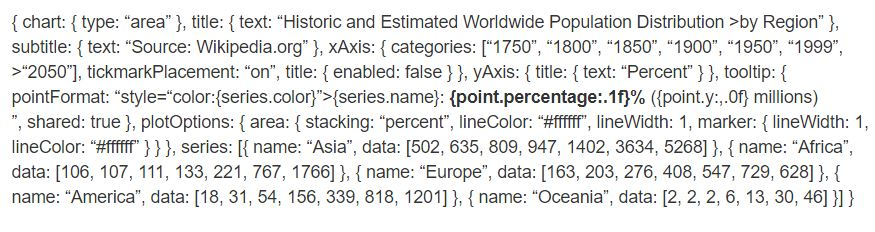``````highchart() %>%
hc_chart(type = "area") %>%
hc_title(text = "Historic and Estimated Worldwide Population Distribution by Region") %>%
hc_subtitle(text = "Source: Wikipedia.org") %>%
hc_xAxis(categories = c("1750", "1800", "1850", "1900", "1950", "1999", "2050"),
tickmarkPlacement = "on",
title = list(enabled = FALSE)) %>%
hc_yAxis(title = list(text = "Percent")) %>%
hc_tooltip(pointFormat = "<span style=\"color:{series.color}\">{series.name}</span>:
<b>{point.percentage:.1f}%</b> ({point.y:,.0f} millions)<br/>",
shared = TRUE) %>%
hc_plotOptions(area = list(
stacking = "percent",
lineColor = "#ffffff",
lineWidth = 1,
marker = list(
lineWidth = 1,
lineColor = "#ffffff"
))
) %>%
hc_add_series(name = "Asia", data = c(502, 635, 809, 947, 1402, 3634, 5268)) %>%
hc_add_series(name = "Africa", data = c(106, 107, 111, 133, 221, 767, 1766)) %>%
hc_add_series(name = "Europe", data = c(163, 203, 276, 408, 547, 729, 628)) %>%
hc_add_series(name = "America", data = c(18, 31, 54, 156, 339, 818, 1201)) %>%
hc_add_series(name = "Oceania", data = c(2, 2, 2, 6, 13, 30, 46))``````While comparing, you can see each of the blocks of javascript code converted into R functions pipelined to each other. We can look at few arguments like hctooltip's pointFormat in the format used as same in Javascript code you can look into details in this.

### Highstocks

Highstocks are charts for Financial and time series analysis, it works well with the `quamtmod` library, and it's easy to chart symbols, and then you can add more series using `hc_add_series`.

``````x <- getSymbols("GOOG", auto.assign = FALSE)

hchart(x)``````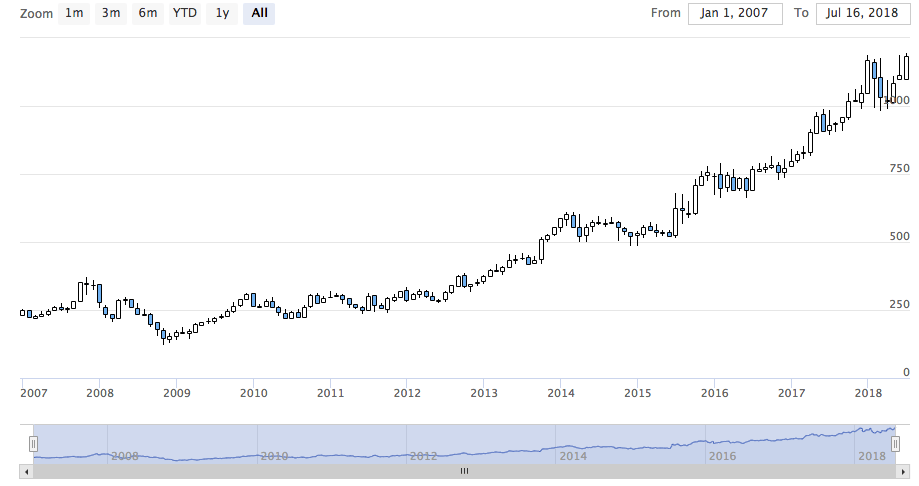As in the chart, we don't need to add additional code, hchart accommodated with xts object very efficiently providing a dynamic snapshot of the data. You can use the zoom functionality to drill down the data in smaller chunks for better analysis.

``````y <- getSymbols("AMZN", auto.assign = FALSE)

highchart(type = "stock") %>%As you can see, the visualization is adjusting the large volume of data very efficiently.

Do try the stock with different xts objects.

### Highmaps

The easiest way to chart a map with highcharter is using an `hcmap` function. Select a URL from the highmaps collection and use the URL as a map in hcmap function. This will download the map and create an object using the info as a mapdata argument.

Let's plot India's map.

``````hcmap("https://code.highcharts.com/mapdata/countries/in/in-all.js")%>%
hc_title(text = "India")``````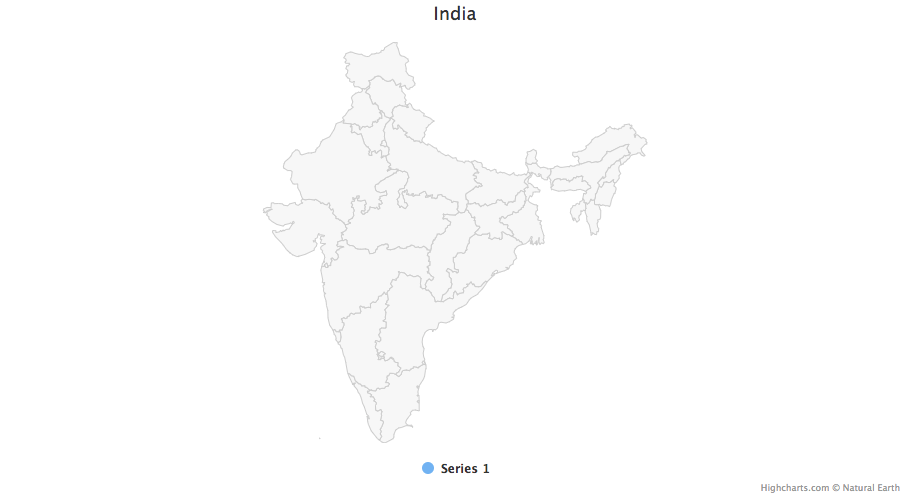Well, that's a plain map what about converting it into choropleths.

Every map data downloaded from highcharts map collection have keys to join data. There are 2 functions to help to know what are the regions coded to know how to join the map and data:

• get_data_from_map: Get the properties for each region in the map, as the keys from the map data.
``````mapdata <- get_data_from_map(download_map_data("https://code.highcharts.com/mapdata/countries/in/in-all.js"))

glimpse(mapdata)``````
``````## Observations: 34
## Variables: 20
## \$ `hc-middle-x` <dbl> 0.65, 0.59, 0.50, 0.56, 0.46, 0.46, 0.51, 0.59, ...
## \$ `hc-middle-y` <dbl> 0.81, 0.63, 0.74, 0.38, 0.64, 0.51, 0.34, 0.41, ...
## \$ `hc-key`      <chr> "in-py", "in-ld", "in-wb", "in-or", "in-br", "in...
## \$ `hc-a2`       <chr> "PY", "LD", "WB", "OR", "BR", "SK", "CT", "TN", ...
## \$ labelrank     <chr> "2", "2", "2", "2", "2", "2", "2", "2", "2", "2"...
## \$ hasc          <chr> "IN.PY", "IN.LD", "IN.WB", "IN.OR", "IN.BR", "IN...
## \$ `alt-name`    <chr> "Pondicherry|Puduchcheri|PondichÃÂ©ry", "Ã\u008dl...
## \$ `woe-id`      <chr> "20070459", "2345748", "2345761", "2345755", "23...
## \$ fips          <chr> "IN22", "IN14", "IN28", "IN21", "IN34", "IN29", ...
## \$ `postal-code` <chr> "PY", "LD", "WB", "OR", "BR", "SK", "CT", "TN", ...
## \$ name          <chr> "Puducherry", "Lakshadweep", "West Bengal", "Ori...
## \$ country       <chr> "India", "India", "India", "India", "India", "In...
## \$ `type-en`     <chr> "Union Territory", "Union Territory", "State", "...
## \$ region        <chr> "South", "South", "East", "East", "East", "East"...
## \$ longitude     <chr> "79.7758", "72.7811", "87.7289", "84.4341", "85....
## \$ `woe-name`    <chr> "Puducherry", "Lakshadweep", "West Bengal", "Ori...
## \$ latitude      <chr> "10.9224", "11.2249", "23.0523", "20.625", "25.6...
## \$ `woe-label`   <chr> "Puducherry, IN, India", "Lakshadweep, IN, India...
## \$ type          <chr> "Union Territor", "Union Territor", "State", "St...``````
``````#population state wise
pop = as.data.frame(c(84673556, 1382611, 31169272, 103804637, 1055450, 25540196, 342853, 242911, 18980000, 1457723, 60383628, 25353081, 6864602,
12548926, 32966238, 61130704, 33387677, 64429, 72597565, 112372972, 2721756, 2964007, 1091014, 1980602, 41947358, 1244464,
27704236, 68621012, 607688, 72138958, 3671032, 207281477, 10116752,91347736))

state= mapdata%>%
select(`hc-a2`)%>%
arrange(`hc-a2`)

State_pop = as.data.frame(c(state, pop))
names(State_pop)= c("State", "Population")

hcmap("https://code.highcharts.com/mapdata/countries/in/in-all.js", data = State_pop, value = "Population",
joinBy = c("hc-a2", "State"), name = "Fake data",
dataLabels = list(enabled = TRUE, format = '{point.name}'),
borderColor = "#FAFAFA", borderWidth = 0.1,
tooltip = list(valueDecimals = 0))``````Do some experiments with hc_add_series in the maps and choropleths. For some details and examples visit here.

### PlugIns

Now let's try some plugins provided by highcharter like grouping, drill-downs, downloading, printing data, and some awesome themes.

Let's group data of mpg dataset, here for better visualization we created a list which categories data according to the manufacturer.

``````data(mpg, package = "ggplot2")

mpgg <- mpg %>%
filter(class %in% c("suv", "compact", "midsize")) %>%
group_by(class, manufacturer) %>%
summarize(count = n())

categories_grouped <- mpgg %>%
group_by(name = class) %>%
do(categories = .\$manufacturer) %>%
list_parse()

highchart() %>%
hc_xAxis(categories = categories_grouped) %>%
hc_add_series(data = mpgg, type = "bar", hcaes(y = count, color = manufacturer),
showInLegend = FALSE)``````Let's create a chart which drills down to another chart for better analysis. You can understand the code more efficiently if you understand lists in R.

``````df <- data_frame(
name = c("Animals", "Fruits", "Cars"),
y = c(5, 2, 4),
drilldown = tolower(name)
)

ds <- list_parse(df)
names(ds) <- NULL

hc <- highchart() %>%
hc_chart(type = "column") %>%
hc_title(text = "Basic drilldown") %>%
hc_xAxis(type = "category") %>%
hc_legend(enabled = FALSE) %>%
hc_plotOptions(
series = list(
boderWidth = 0,
dataLabels = list(enabled = TRUE)
)
) %>%
name = "Things",
colorByPoint = TRUE,
data = ds
)

dfan <- data_frame(
name = c("Cats", "Dogs", "Cows", "Sheep", "Pigs"),
value = c(4, 3, 1, 2, 1)
)

dffru <- data_frame(
name = c("Apple", "Organes"),
value = c(4, 2)
)

dfcar <- data_frame(
name = c("Toyota", "Opel", "Volkswage"),
value = c(4, 2, 2)
)

second_el_to_numeric <- function(ls){

map(ls, function(x){
x[] <- as.numeric(x[])
x
})

}

dsan <- second_el_to_numeric(list_parse2(dfan))

dsfru <- second_el_to_numeric(list_parse2(dffru))

dscar <- second_el_to_numeric(list_parse2(dfcar))

hc %>%
hc_drilldown(
allowPointDrilldown = TRUE,
series = list(
list(
id = "animals",
data = dsan
),
list(
id = "fruits",
data = dsfru
),
list(
id = "cars",
data = dscar
)
)
)``````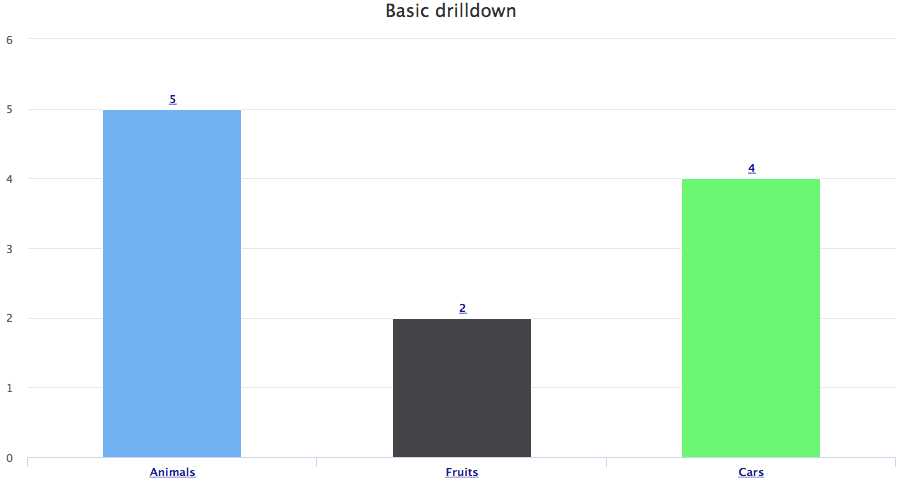Let's apply Drill down on other charts.

``````tm <- pokemon %>%
mutate(type_2 = ifelse(is.na(type_2), paste("only", type_1), type_2),
type_1 = type_1) %>%
group_by(type_1, type_2) %>%
summarise(n = n()) %>%
ungroup() %>%
treemap::treemap(index = c("type_1", "type_2"),
vSize = "n", vColor = "type_1")``````
.``````tm\$tm <- tm\$tm %>%
tbl_df() %>%
left_join(pokemon %>% select(type_1, type_2, color_f) %>% distinct(), by = c("type_1", "type_2")) %>%
left_join(pokemon %>% select(type_1, color_1) %>% distinct(), by = c("type_1")) %>%
mutate(type_1 = paste0("Main ", type_1),
color = ifelse(is.na(color_f), color_1, color_f))

highchart() %>%
layoutAlgorithm = "squarified")``````Let's add the functionality of exporting data.

``````pokemon%>%
count(type_1)%>%
arrange(n)%>%
hchart(type = "bar", hcaes(x = type_1, y = n, color = type_1))%>%
hc_exporting(enabled = TRUE)``````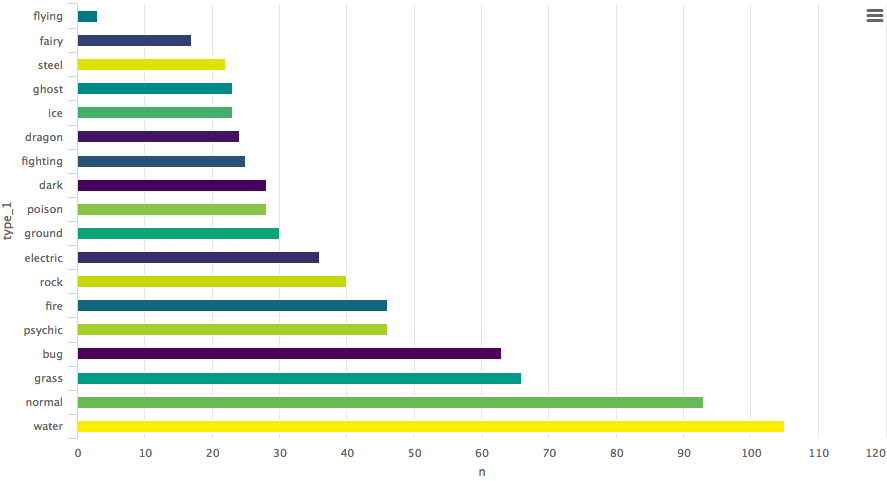At last, we add some themes.
``````pokemon%>%
count(type_1)%>%
arrange(n)%>%
hchart(type = "bar", hcaes(x = type_1, y = n, color = type_1))%>%
hc_exporting(enabled = TRUE)%>%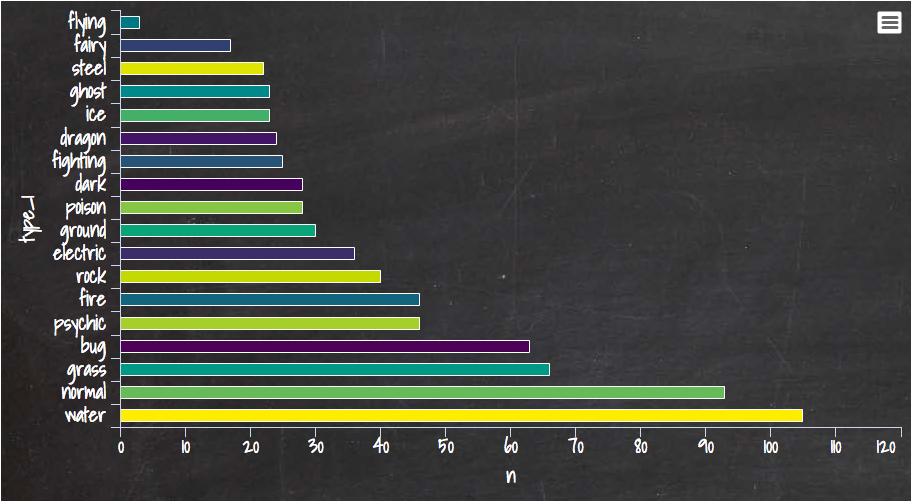You can learn a lot from here, Its a complete library for Highcharter. I am also sharing one of my favorite graph created on weather dataset, here one of the interesting argument `polar` is used by setting the argument to TRUE the whole story is transformed.

``````data("weather")

x <- c("Min", "Mean", "Max")
y <- sprintf("{point.%s}", c("min_temperaturec", "mean_temperaturec", "max_temperaturec"))
tltip <- tooltip_table(x, y)

hchart(weather, type = "columnrange",
hcaes(x = date, low = min_temperaturec, high = max_temperaturec,
color = mean_temperaturec)) %>%
hc_chart(polar = TRUE) %>%
hc_yAxis( max = 30, min = -10, labels = list(format = "{value} C"),
showFirstLabel = FALSE) %>%
hc_xAxis(
title = list(text = ""), gridLineWidth = 0.5,
labels = list(format = "{value: %b}")) %>%
hc_tooltip(useHTML = TRUE, pointFormat = tltip,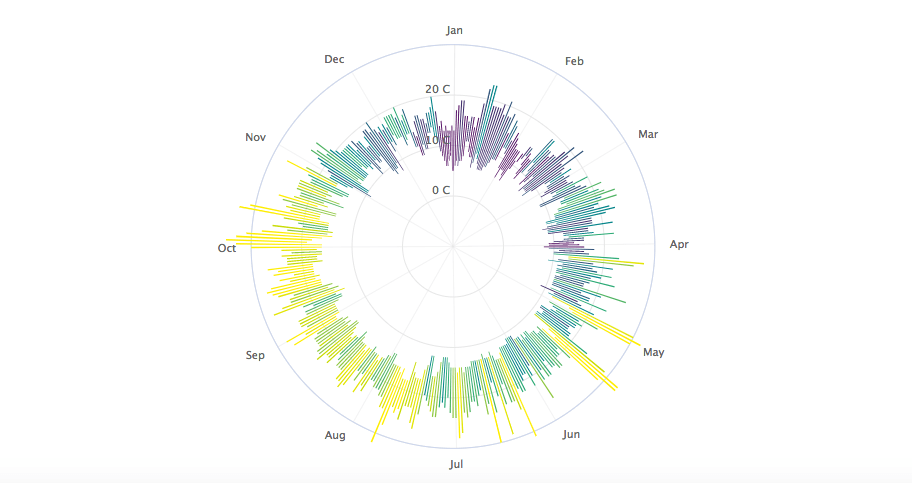If you would like to learn more about Data Visualization in R, take DataCamp's Data Visualization with ggplot2 (Part 1) course and check out our R Formula Tutorial.

### .css-1531qan{-webkit-text-decoration:none;text-decoration:none;color:inherit;}Introduction to Data Visualization with ggplot2

BeginnerSkill Level
4 hr
115.8K
Learn to produce meaningful and beautiful data visualizations with ggplot2 by understanding the grammar of graphics.
See Details
Start Course

### Interactive Data Visualization with plotly in R

BeginnerSkill Level
4 hr
10.4K
Learn how to use plotly in R to create interactive data visualizations to enhance your data storytelling.

### Visualization Best Practices in R

BeginnerSkill Level
4 hr
15K
Learn to effectively convey your data with an overview of common charts, alternative visualization types, and perception-driven style enhancements.
See More
Related### Free Access Week | Aug 28 – Sept 3

Access DataCamp's entire platform for free, including all 440+ courses, for an entire week. No catch, no credit card required—just unlimited learning for anyone with internet access.

Will Rix

5 min### 11 Data Visualization Techniques for Every Use-Case with Examples

Discover the most popular analyses, techniques and tools to master the art of data visualization wizard

### How to Choose The Right Data Science Bootcamp in 2023 (With Examples)

Learn everything about data science bootcamps, including a list of top programs to kickstart your career.### DataCamp Portfolio Challenge: Win \$500 Publishing Your Best Work

Win up to \$500 by building a free data portfolio with DataCamp Portfolio.DataCamp Team

5 min

### Chroma DB Tutorial: A Step-By-Step Guide

With Chroma DB, you can easily manage text documents, convert text to embeddings, and do similarity searches.

### Introduction to Non-Linear Model and Insights Using R

Uncover the intricacies of non-linear models in comparison to linear models. Learn about their applications, limitations, and how to fit them using real-world data sets.

Somil Asthana

17 min

See MoreSee More